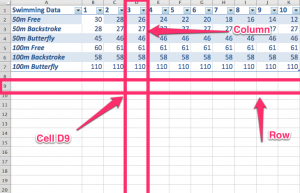June 5

# Excel 101: Starting with Spreadsheets

Watch the following video looking at the basic parts of a spreadsheet.

Key ideas:

• A column is the part of a spreadsheet or table going down the page. This is indicated by a column header that is a letter (eg, A, B, C)
• A row goes across the page, and is indicated by a number (1, 2, 3)
• A cell is the intersection of a row and a column, where data is stored. This is referred to by its cell address or cell reference. (eg, A1, B3, G5)
• Cell references are used within formulas.See if you can figure out the cell references from the following image:Basic Formulas =B1+B2

Key ideas:

• You can click on the cells to enter their cell reference in the formula.
• You can copy down formulas and they will copy the cell references down (these are called relative formulas)
• Any simple mathematical function can be used within a spreadsheet =B1+B2, =B1-B2, =B1/B2, =B1*B2 (multiplication) , =B1-B2+3*B4-B1/2

Key ideas:

• There are a whole heap of pre-defined formulas. Some examples are =Max() =Min() =Average() =Sum()
• You can refer to a range of cells from the top left to the bottom right cell: eg:

Absolute and relative Cell References

Key points:

• Relative cell references are references that are relative to where the answer is: ie, typing in =b1 in B2 actually just means “the cell directly above me”
• Absolute cell references are absolutely where you say that they are. ie, if you type in =\$b\$1 in the cell, it means: “no matter where you move me to, always equal what is in B1”
• This is an advanced technique that you can use with things like the Rank() formula.

Posted June 5, 2013 by shortcomp in category IPT